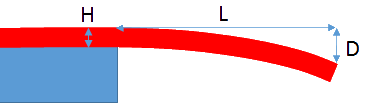## Modulus from Bending

### Quick Start

Suppose you have a thin sample of material which is much too weak to put into a tensile tester to measure its modulus, and you have no access to a fancy nanoindenter. No problem. Cut out a piece of thickness H and estimate its density, then let a length L hang freely in space. Now measure the distance, D, the end has drooped. Put the values into the app and you have the modulus.

### Modulus from Bending

Length L mm
Distance D mm
Density ρ g/cc
Thickness H μm
Modulus E GPa

Sometimes you need to measure the modulus of a thin film. Putting it into a tensile tester is not practical. Those who have access to a nanoindenter might be able to get a good value by analysing the loading and unloading curves. For the rest of us a really simple yet accurate method can be used. Simply measure how much bending there is from a strip of a known thickness and length!The formula is simple. The modulus, E, for a film of thickness H and density ρ which bends by distance D over a length L is given by:

E=(1.3L^4ρg)/(H²D)

Obviously any curl in the sample before measurement adds confusion. If you can't obtain a curl-free sample, measure it both ways up and take an average! Note the L4 dependency; this means that you must measure L with some accuracy. But it also means that you have a lot of freedom to get a nice measurable droop simply by changing L. It's also a good idea to measure D at different L values to increase your overall accuracy.

If you prefer to measure the droop of a sample supported at both ends, use L as the half-width and divide the calculated E by 8.